# Propagation of uncertainties for a function of observables

This is a simpler presentation of a well-known result, see e.g. wikipedia.
Alex Read, Department of Physics, University of Oslo, December, 2020
We want to estimate the uncertainty of a function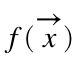of a set of n observables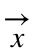with associated uncertainties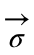. We assume that the observablesare sampled from normal distributions that are narrow relative to the form of the function and thus we approximate the function with its Taylor-series expansion, keeping only the lowest-order derivatives,
where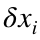represents the Gaussian-distributed deviation from the true mean, which is unknown, but estimated by the observed value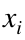. In other words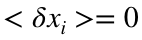and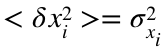.
The uncertainty in f is estimated as the square root of its mean variance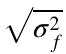, where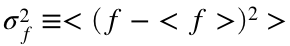. We replace f with the series approximation above and take advantage of that in this approximation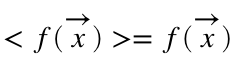. In addition, we write the dot product as an explicit sum, taking care to have separate indices for the terms in the square of the dot product, obtaining. This can be re-written as the sum of 2 terms:.

## Multiple uncorrelated observables

If there is no correlation between the observables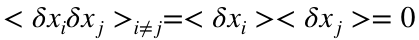and the second term above is 0. Since the variance ofis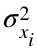, the final and familiar result is. In other words, uncorrelated contributions to the total uncertainty of a function of observables are to be added in quadrature.

## A single observable

If there is only a single observable we have. This can be visualized in the illustration below. The function is approximated by its slope at the point of the observation. The variance on the x-axis is transformed through the slope to the variance on the y-axis, i.e. f.
%plot an illustrative parabola
xwide = linspace(0,5,1000);
ywide = 1*xwide.^2 + 2*xwide + 3;
figure
subplot(2,2,2)
plot(xwide,ywide,'k-')
%draw a tangent to a point (e.g. 3.5)
hold on
xpObs = 3.5;
ypObs = 1*xpObs.^2 + 2*xpObs + 3;
plot(xpObs,ypObs,'k.','MarkerSize',10)
dypObs = 2*xpObs+2;
plot(xwide,dypObs*(xwide-xpObs)+ypObs,'k:','LineWidth',1)
xlabel('x'), ylabel('y')
title('f(x) and tangent at x_0')
ca2 = gca;
grid on
% Plot the pdf of x
sigma_x = 0.3;
subplot(2,2,4)
hx = histogram(normrnd(xpObs,sigma_x,10000,1));
hx.Normalization = 'pdf';
ca = gca;
ca.XLim = ca2.XLim;
xlabel('x'), ylabel('Probability')
title('pdf of x_0')
grid on
%histogram the transformed distribution on the y-axis
subplot(2,2,1)
hy = histogram(normrnd(ypObs,0.3*dypObs,10000,1));
hy.Orientation = 'horizontal';
hy.Normalization = 'pdf';
ca = gca;
ca.YLim = ca2.YLim;
ylabel('y'), xlabel('Probability')
title('pdf of f(x_0)')
grid on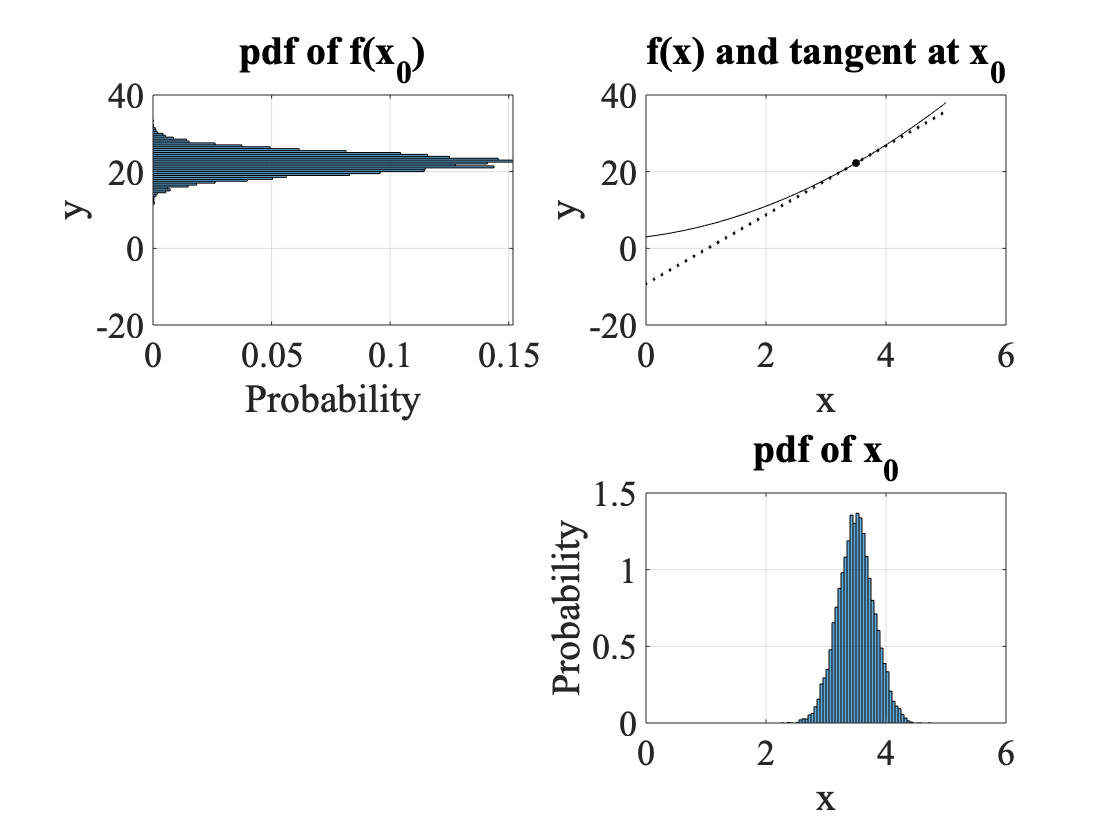In the upper right plot we see the function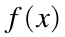shown as a solid curve and the tangent at a particular point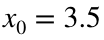shown as a dotted line. In the lower right plot we see the pdf of x (a normal distribution) given the observation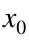and the uncertainty on,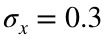. In the upper left plot we see the pdf of(also a normal distribution) givenand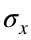. The ratio of the spread (RMS) of f to that of x is given by the slope of the tangent, i.e..

### Example with multiple uncorrelated observables: Volume of a box

We measure the height h, width w, and depth d of a box with uncertainties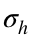,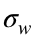, and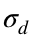, respectively and want to estimate the volume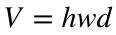and its uncertainty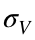. Assuming the measurements are uncorrelated we calculate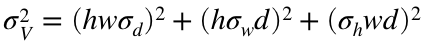. By the way, by dividing both sides of this by the volume we obtain an elegant expression for the relative uncertainty on the volume: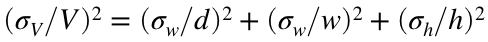.
Let's study a numerical example: Suppose we measure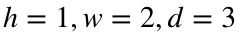cm with relative uncertainties 1, 2, and 3%. The volume and its uncertainty are calculated below.
h = 1; w = 2; d = 3;
dhRel = 0.01; dwRel = 0.01; ddRel = 0.03;
V = h * w * d;
dVrel = sqrt(dhRel^2+dwRel^2+ddRel^2);
dV = dVrel * V;
fprintf('V = %.3f +- %.3f cc\n',V,dV)
V = 6.000 +- 0.199 cc
fprintf('Relative uncertainty on V = %.1f %%\n',dVrel*100)
Relative uncertainty on V = 3.3 %
We see that the relative uncertainty on d dominates the relative uncertainty on V.
Let's create an ensemble of Monte Carlo experiments of the measurement, by generating (uncorrelated!) random values of h, w, and d according to their uncertainties, and calculate the mean and standard deviation of V.
N = 10000; % Number of Monte Carlo experiments
h = normrnd(h,h*dhRel,N,1);
w = normrnd(w,w*dwRel,N,1);
d = normrnd(d,d*ddRel,N,1);
V = h.*w.*d;
figure
hist = histogram(V);
xlabel('Volume (cc)')
ylabel('Measurements/bin')
title('Histogram of Monte Carlo measurements of V')
meanV = mean(V); sigmaV = std(V);
fprintf('Mean and std.dev. of V = %.3f +- %.3f',mean(V),std(V))
Mean and std.dev. of V = 6.001 +- 0.199
x = linspace(hist.BinEdges(1),hist.BinEdges(end),100);
y = normpdf(x,meanV,sigmaV)*N*hist.BinWidth;
hold on
plot(x,y,'r-')
legend('Measurements','Normal distr.')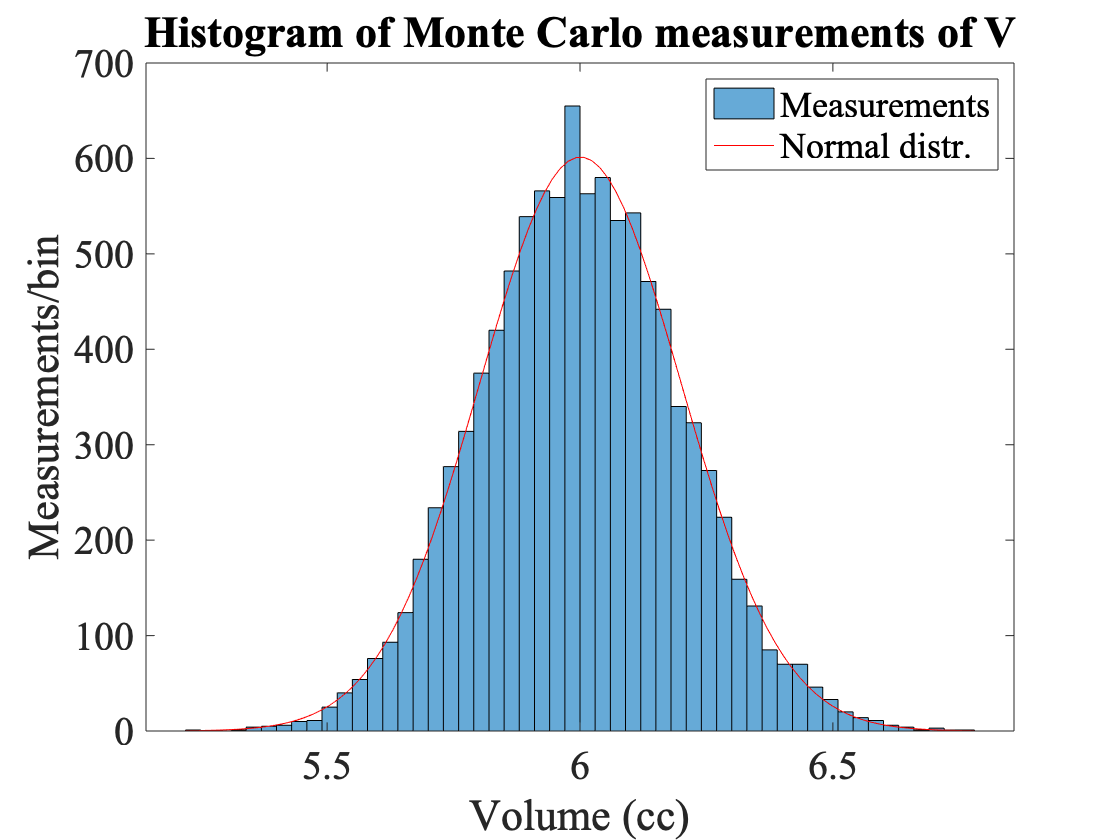With 10k Monte Carlo experiments we see that the analytic and numerical propagations of uncertainty are consistent to several significant digits. We also see that the distribution of measured volumes is well described by the normal distribution.

## Correlated observables

There are sizeable correlations between observables in many experiments, i.e. the covariances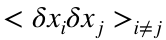are not zero but rather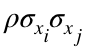, where the correlation coefficient ρ can take on values anywhere between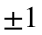. For example, the slope and intercept of a straight line fitted to data will tend to be correlated (unless the x-axis is chosen carefully). In this case we have.

### Example: The uncertainty on y at a given x of a fitted straight line

The equation of a line can be written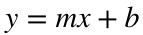. The uncertainty on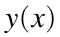, keeping the correlation terms, is. Here we see that the uncertainty on y at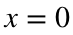is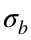, which is natural since b is the y-intercept of the line. It turns out that the covariance is proportional to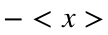, and that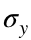is minimized at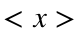.
These features we can see in the plot below, where a straight line is fitted to an artificial dataset.
Generate 10 Monte Carlo or "toy" datapoints with the same normal uncertainty at each point.
x = linspace(1,10,10);
mmodel = 0.25; bmodel = 2; sigma_y = 0.5;
y0 = mmodel*x+bmodel; % The modell
y = normrnd(y0,sigma_y); % The fake data generated from the model
Plot the data as points with error bars.
figure(1)
hold off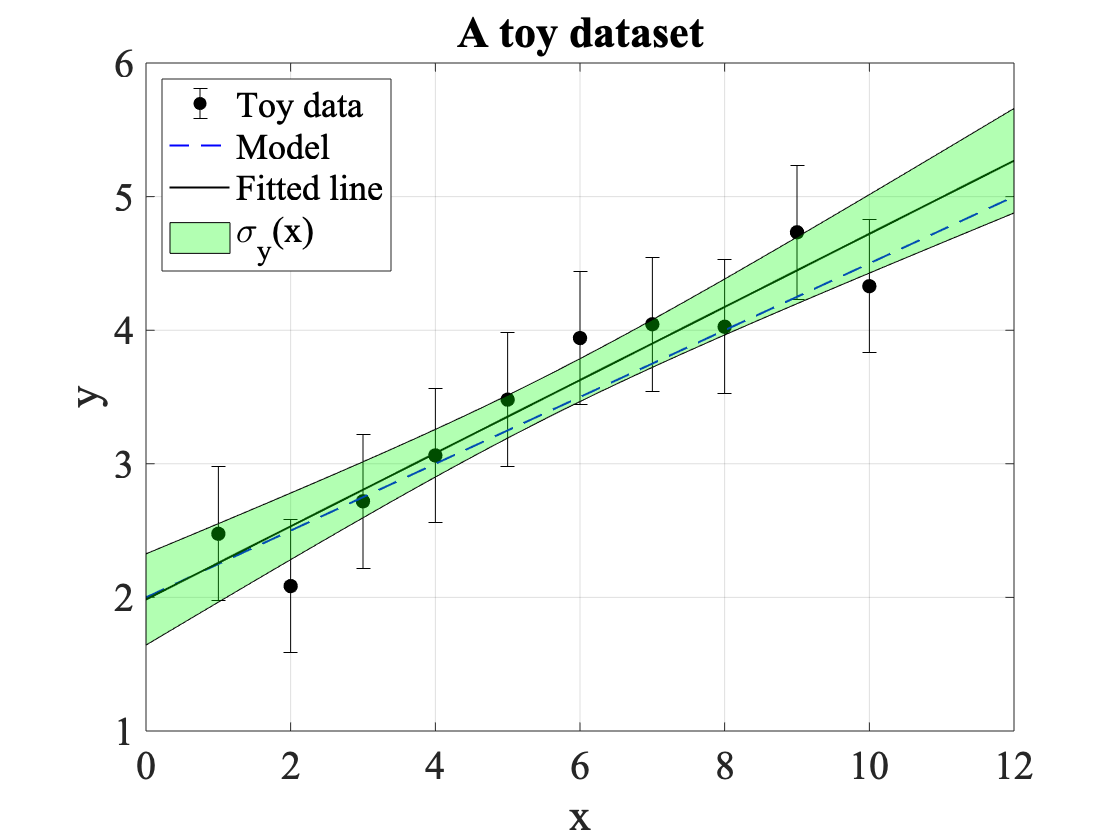errorbar(x,y,ones(length(y),1)*sigma_y,'k.','MarkerSize',20)
hold on
xlabel('x'), ylabel('y')
title('A toy dataset')
Fit a line to the data by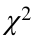minimization and extract the slope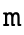and intercept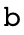.
% Define the model to perform a chi-squared fit of
model = @(p,x) x*p(1)+p(2); %+ x.^2*p(3);
dy = sigma_y*ones(size(y));
% Rough guess of the parameter values to guide minimization
guess = [1,1];
[p,perrors,chisqmin,exitflag,C ] = fitchisq(x,y,dy,model,guess);
m=p(1);
b=p(2);
Extract the variances and covariance from the covariance (or error) matrix C. The diagonal elements of C are the variances of the parameters. Non-zero off-diagonal elements indicate a covariance between the parameters.
dm2 = C(1,1); % Error in the slope
db2 = C(2,2); % Error in the intercept
dmb = C(1,2); % Covariance
fprintf('Covariance of fitted (m,b) = %.3f\n',dmb)
Covariance of fitted (m,b) = -0.017
fprintf('Correlation coefficient (m,b) = %.3f\n',dmb/sqrt(dm2*db2))
Correlation coefficient (m,b) = -0.886
Evaluate and plot the model and the fitted result.
xwide = linspace(0,12,100);
yfit = polyval(p,xwide);
y0wide = mmodel*xwide+bmodel;
plot(xwide,y0wide,'b--','LineWidth',1)
plot(xwide,yfit,'k','LineWidth',1)
Calculate and plot the error in y as function of x, represented by a green band.
dywide = sqrt(xwide.^2*dm2 + db2 + 2*xwide*dmb);
% Plot error in y as function of x as a transparent green band
f=fill([xwide flip(xwide)],[yfit-dywide flip(yfit+dywide)],'g');
f.FaceAlpha=0.3;
% Put the grid on so we can see the y and x intercepts
grid on
legend('Toy data','Model','Fitted line','\sigma_y(x)','Location','NorthWest')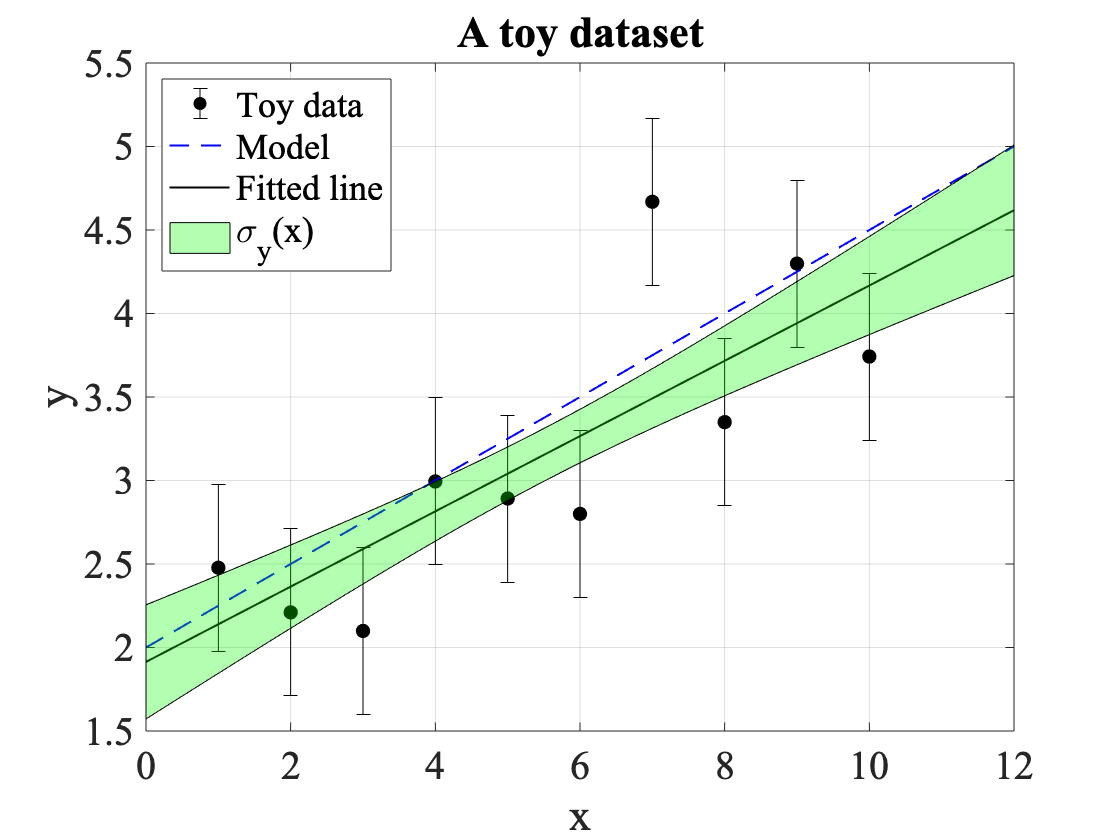We see that the fitted line ressembles the model line, the data points are spread around the model line, and the fitted line tends to be within the predicted variance of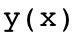(the green band). We also see that the variance band is narrowest in the middle of the data points.
If the error band of the line has any value, then it should be consistent with the root-mean-square (RMS) of the lines fitted to an ensemble of toys!
N = 1000;
yfit = zeros(N,length(y));
for i=1:N
y = normrnd(y0,sigma_y);
[p,perrors,chisqmin,exitflag,C ] = fitchisq(x,y,dy,model,guess);
yfit(i,:) = polyval(p,x);
end
ymean = mean(yfit,1);
ystd = std(yfit,1);
figure
plot(xwide,y0wide,'b-','LineWidth',1)
hold on
errorbar(x,ymean,ystd,'k.','MarkerSize',10)
% Plot error in y as function of x as a transparent green band
f=fill([xwide flip(xwide)],[y0wide-dywide flip(y0wide+dywide)],'g');
f.FaceAlpha=0.3;
legend('Model','Fitted toys','Model variance','Location','NorthWest')
xlabel('x'), ylabel('y'),title('Mean and RMS of many fitted toys')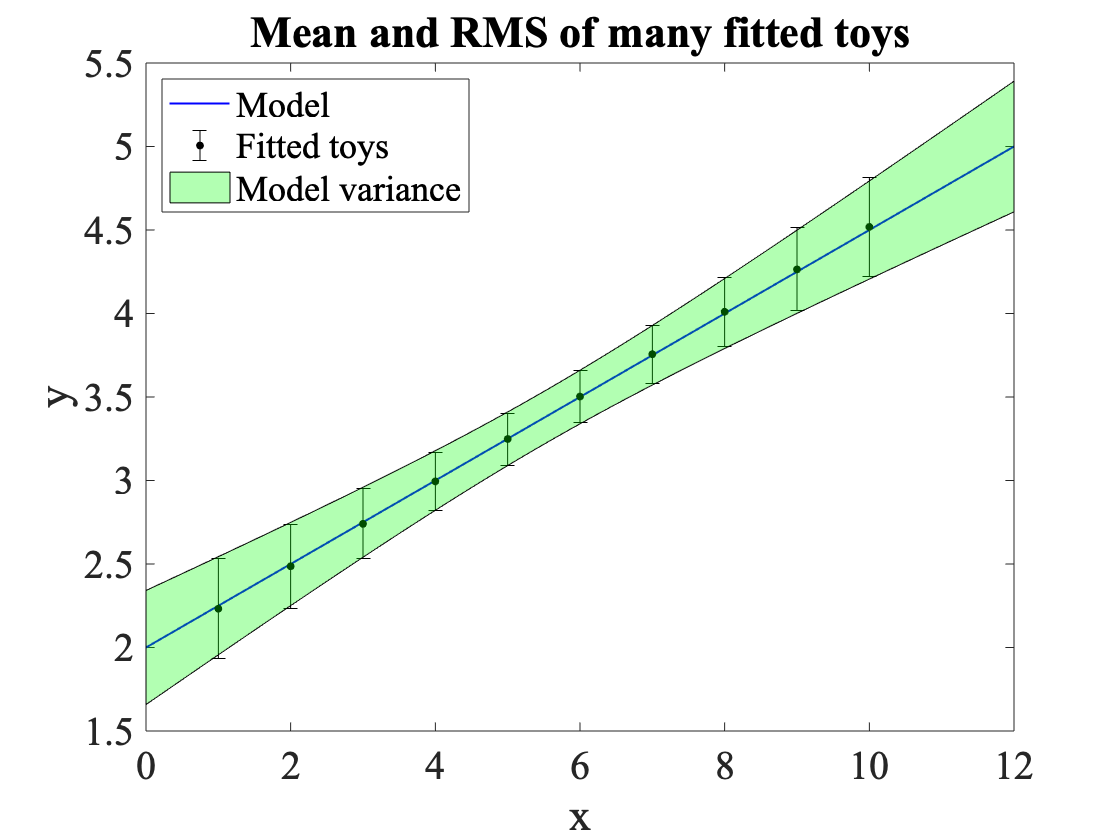We see that the mean and RMS of the toys are consistent with the model and the predicted variance in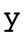, including the covariance term. The parabolic shape of the band indicates, corresponding to the negative covariance, that an upward flucutation of the slope tends to give a downward fluctuation of the y-intercept, and vis-versa.

## Summary

We have derived formulae for error propagation for functions of observables under the condition that the function is approximately linear over the span of the uncertainties on the observables.
We have seen where the rule-of-thumb to combine uncorrelated uncertainties in quadrature comes from.
We have studied examples of both uncorrelated and correlated observables and reproduced the results of the formulae with Monte Carlo "toy" experiments.